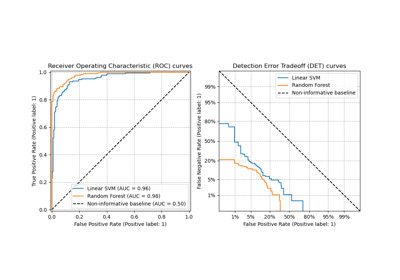# sklearn.metrics.det_curve¶

sklearn.metrics.det_curve(y_true, y_score, pos_label=None, sample_weight=None)[source]

Compute error rates for different probability thresholds.

Note

This metric is used for evaluation of ranking and error tradeoffs of a binary classification task.

Read more in the User Guide.

New in version 0.24.

Parameters:
y_truendarray of shape (n_samples,)

True binary labels. If labels are not either {-1, 1} or {0, 1}, then pos_label should be explicitly given.

y_scorendarray of shape of (n_samples,)

Target scores, can either be probability estimates of the positive class, confidence values, or non-thresholded measure of decisions (as returned by “decision_function” on some classifiers).

pos_labelint, float, bool or str, default=None

The label of the positive class. When pos_label=None, if y_true is in {-1, 1} or {0, 1}, pos_label is set to 1, otherwise an error will be raised.

sample_weightarray-like of shape (n_samples,), default=None

Sample weights.

Returns:
fprndarray of shape (n_thresholds,)

False positive rate (FPR) such that element i is the false positive rate of predictions with score >= thresholds[i]. This is occasionally referred to as false acceptance probability or fall-out.

fnrndarray of shape (n_thresholds,)

False negative rate (FNR) such that element i is the false negative rate of predictions with score >= thresholds[i]. This is occasionally referred to as false rejection or miss rate.

thresholdsndarray of shape (n_thresholds,)

Decreasing score values.

DetCurveDisplay.from_estimator

Plot DET curve given an estimator and some data.

DetCurveDisplay.from_predictions

Plot DET curve given the true and predicted labels.

DetCurveDisplay

DET curve visualization.

roc_curve

Compute Receiver operating characteristic (ROC) curve.

precision_recall_curve

Compute precision-recall curve.

Examples

>>> import numpy as np
>>> from sklearn.metrics import det_curve
>>> y_true = np.array([0, 0, 1, 1])
>>> y_scores = np.array([0.1, 0.4, 0.35, 0.8])
>>> fpr, fnr, thresholds = det_curve(y_true, y_scores)
>>> fpr
array([0.5, 0.5, 0. ])
>>> fnr
array([0. , 0.5, 0.5])
>>> thresholds
array([0.35, 0.4 , 0.8 ])


## Examples using sklearn.metrics.det_curve¶